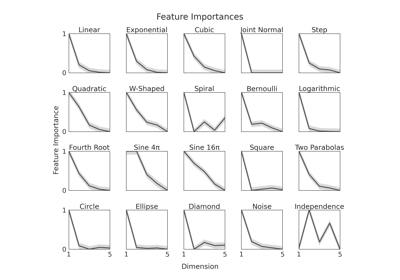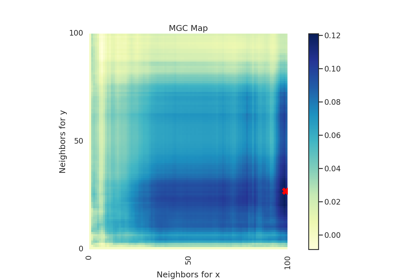# KMERF¶

class hyppo.independence.KMERF(forest='regressor', ntrees=500, **kwargs)

Kernel Mean Embedding Random Forest (KMERF) test statistic and p-value.

The KMERF test statistic is a kernel method for calculating independence by using a random forest induced similarity matrix as an input, and has been shown to have especially high gains in finite sample testing power in high dimensional settings 1.

Parameters

Notes

A description of KMERF in greater detail can be found in 1. It is computed using the following steps:

Let $$x$$ and $$y$$ be $$(n, p)$$ and $$(n, 1)$$ samples of random variables $$X$$ and $$Y$$.

• Run random forest with $$m$$ trees. Independent bootstrap samples of size $$n_{b} \leq n$$ are drawn to build a tree each time; each tree structure within the forest is denoted as $$\phi_w \in \mathbf{P}$$, $$w \in \{ 1, \ldots, m \}$$; $$\phi_w(x_i)$$ denotes the partition assigned to $$x_i$$.

• Calculate the proximity kernel:

$\mathbf{K}^{\mathbf{x}}_{ij} = \frac{1}{m} \sum_{w = 1}^{m} I(\phi_w(x_i) = \phi_w(x_j))$

where $$I(\cdot)$$ is the indicator function for how often two observations lie in the same partition.

• Compute the induced kernel correlation: Let

$\begin{split}\mathbf{L}^{\mathbf{x}}_{ij}= \begin{cases} \mathbf{K}^{\mathbf{x}}_{ij} - \frac{1}{n-2} \sum_{t=1}^{n} \mathbf{K}^{\mathbf{x}}_{it} - \frac{1}{n-2} \sum_{s=1}^{n} \mathbf{K}^{\mathbf{x}}_{sj} + \frac{1}{(n-1)(n-2)} \sum_{s,t=1}^{n} \mathbf{K}^{\mathbf{x}}_{st} & \mbox{when} \ i \neq j \\ 0 & \mbox{ otherwise} \end{cases}\end{split}$
• Then let $$\mathbf{K}^{\mathbf{y}}$$ be the Euclidean distance induced kernel, and similarly compute $$\mathbf{L}^{\mathbf{y}}$$ from $$\mathbf{K}^{\mathbf{y}}$$. The unbiased kernel correlation equals

$\mathrm{KMERF}_n(\mathbf{x}, \mathbf{y}) = \frac{1}{n(n-3)} \mathrm{tr} \left( \mathbf{L}^{\mathbf{x}} \mathbf{L}^{\mathbf{y}} \right)$

The p-value returned is calculated using a permutation test using hyppo.tools.perm_test.

References

1(1,2)

Cencheng Shen, Sambit Panda, and Joshua T. Vogelstein. Learning Interpretable Characteristic Kernels via Decision Forests. arXiv:1812.00029 [cs, stat], September 2020. arXiv:1812.00029.

Methods Summary

 Helper function that calculates the KMERF test statistic. KMERF.test(x, y[, reps, workers, random_state]) Calculates the KMERF test statistic and p-value.

KMERF.statistic(x, y)

Helper function that calculates the KMERF test statistic.

Parameters

x,y (ndarray) -- Input data matrices. x and y must have the same number of samples. That is, the shapes must be (n, p) and (n, 1) where n is the number of samples and p is the number of dimensions.

Returns

stat (float) -- The computed KMERF statistic.

KMERF.test(x, y, reps=1000, workers=1, random_state=None)

Calculates the KMERF test statistic and p-value.

Parameters
• x,y (ndarray) -- Input data matrices. x and y must have the same number of samples. That is, the shapes must be (n, p) and (n, 1) where n is the number of samples and p is the number of dimensions.

• reps (int, default: 1000) -- The number of replications used to estimate the null distribution when using the permutation test used to calculate the p-value.

• workers (int, default: 1) -- The number of cores to parallelize the p-value computation over. Supply -1 to use all cores available to the Process.

Returns

• stat (float) -- The computed KMERF statistic.

• pvalue (float) -- The computed KMERF p-value.

• kmerf_dict (dict) --

Contains additional useful returns containing the following keys:

• feat_importancendarray

An array containing the importance of each dimension

Examples

>>> import numpy as np
>>> from hyppo.independence import KMERF
>>> x = np.arange(100)
>>> y = x
>>> '%.1f, %.2f' % KMERF().test(x, y)[:1]
'1.0, 0.001'


## Examples using hyppo.independence.KMERF¶